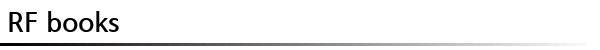조회수 : 2106 도서명 Introduction to Computer Methods for Microwave Circuit Analysis and Design 저자/역자 Dobrowolski 출판사 Artech House 분야 전자파 수치해석 목차/설명 This book presents appropriate parts of microwave circuit theory and the mathematical and numerical methods required for computer-aided analysis and design of linear microwave circuits. Topics discussed include: matrix representations used in CAD, computer aided methods for linear circuit analysis in frequency domain, sensitivity analysis methods, noise analysis methods and noise power sensitivity methods, tolerance analysis, and tolerance design methods. Contents: Preface. Microwave Circuit Matrix Representations. Computer-Aided Analysis of Microwave Circuits. Computer-Aided Sensitivity Analysis of Microwave Circuits. Computer-Aided Noise Analysis of Microwave Circuits. Numerical Methods for Solving Systems of Linear Algebraic Equations. Sparse Matrix Techniques. Sparse Matrix Techniques for Analysis of Microwave Circuits Described by the Connection Scattering Matrix. Tolerance Analysis and Design of Microwave Circuits. Optimization Techniques for Microwave Circuit Design. References. Appendices. Index. 등록자 admin   수정하기
 ▼ 추천/비평(0)
 목록으로 이 책을 보신 분이라면 목차/ 내용추가설명이나 비평,추천/비추천에 대한 의사를 표시해주세요! 해당서적과 관련된 내용이면 어떤 내용이든 상관없습니다. 아이디 :    비밀번호 :